# Phase delay

New for April 2015! After providing an hour's worth of material on analog/RF time delay at GOMACTech 2015 for a tutorial on phased arrays and beam-steering, the Unknown Editor will spread the word on phase delay on this page.

There seems to be a broad misunderstanding about how to determine time delay from S-parameters. Here is an app note that tells you to look at group delay, which will give you misleading results. Microwaves101 will set you straight. Phase delay is what is used in designing time delay units, in order to convert S-parameter data into units of time. This is not complicated calculus like Fourier transforms, it is simple algebra. Not everyone knows this, but phase delay is by definition, time delay, as we will derive below.

## Phase delay definition

Phase delay (a.k.a. time delay) is calculated from the unwrapped transmission phase angle of a network: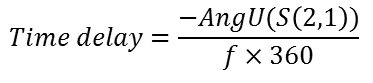When frequency is in GHz, time delay will be in nanoseconds. Angle is in degrees, if it was in radians you would divide by 2xpi, so says Captain Obvious.

Phase delay is the true measure of time delay, which can be proven from the Telegrapher's equations. Behold the image below, it has been used to describe transmission line behavior since the 19th century. The "prime" notation implies that these are incremental values of lumped elements normalized per unit length (Henries per meter, Ohms per meter, Farads per meter and Siemens per meter). All of names of the units are capitalized as they are names of scientists who can be found in our Microwave Hall of Fame (click on their links).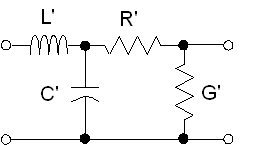Let's start with the equation that exactly describes the propagation constant of a wave along a transmission line: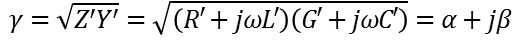(1)

Now we will make the a simplification that is one of the foundations of microwave engineering: for near loss-less transmission lines, (R’<<ωL’ and  G’ << ωC’ ), the propagation constant can be separated into a phase constant and an attenuation constant.

Here is the attenuation constant: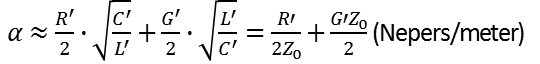(2)

and here is the phase constant: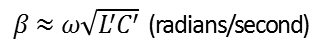(3)

By the way, here is a derivation of that simplification, by Dr. Alex Arsenovic, who offers free microwave analysis software on scikit-rf.org. No, that is not a hair on your screen, it was on the scanner. Perhaps you can send the image out for a DNA test to see just who might have left a hair there... just don't send it  to the FBI or the wrong man will get convicted.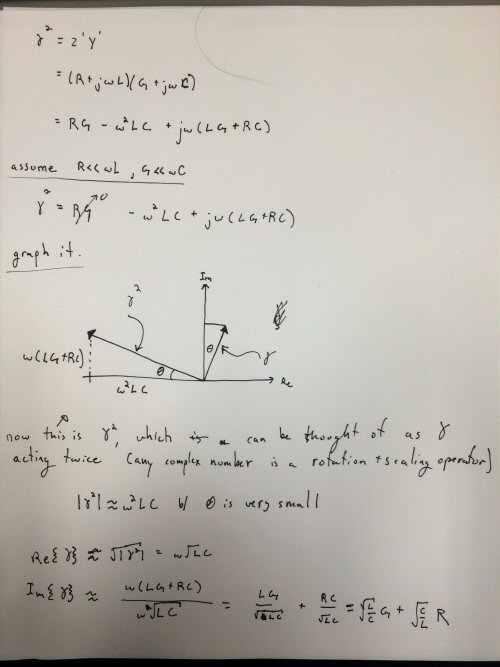The two constants have entirely different effects on the wave as shown below. The phase constant is what defines the periodicity of the sine wave, while the attenuation constant defines an envelope. The plot shows an extremely lossy propagation constant, perhaps it is close the attenuation constant in high voltage transmission lines spanning twenty thousands of miles at 60 Hz (wavelength is 3100 miles so you would see six peaks). Oops, we were supposed to used the metric system here, let me get back to you on how many km that is...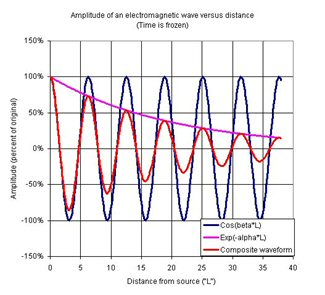Wavelength is distance where phase changes by 2π radians: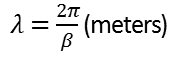(4)

Phase velocity is defined as wavelength multiplied by frequency, which by substitution reveals: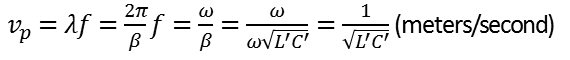(5)

Time delay is defined as length of line divided by phase velocity: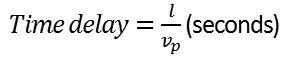(6)

Noting that phase velocity is: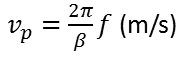(7)

and time delay is length divided by phase velocity: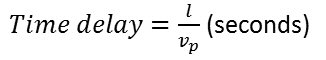(8)

Time delay can be expressed in terms of βl: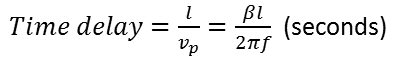(9)

Electrical length is what designer use when they are looking at ideal circuits in a linear simulator. This is a Microwave Office transmission line element with electrical  length ("EL") identified: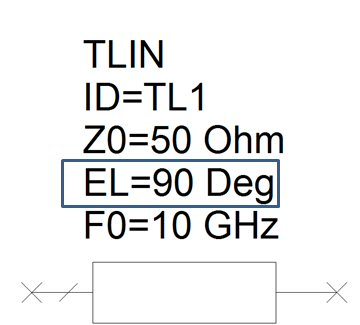Electrical length can be calculated from βl as follows (reference: Microwave Office Knowledge Base):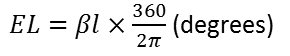(10)

Now we can substitute EL for βl in the time delay equation: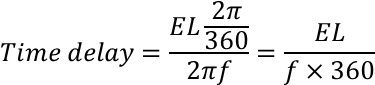(11)

We are almost done.  Note that electrical length of as transmission line is related to the unwrapped phase angle of S21. If your electrical length was 360 degrees, the unwrapped phase angle would be -360 degrees, for example.(12)

One final substitution into the time delay equation and we get the formula for phase delay. Voila!(13)

## Phase delay versus group delay

More text coming soon. No kidding! for now, please enjoy the following table:

 Phase delay Group delay Used by TDU designers Used by filter designers True measurement of time delay calculated from transmission phase Inaccurate measurement of time delay calculated from transmission phase Often quite painful to calculate Easy to calculate Absolute phase delay may be ambiguous, especially if low frequency data are missing. True relative time delay of states can be calculated unambiguously Ambiguous results are observed when frequency increment is changed Subject to two types of aliasing Subject to one type of aliasing Relatively insensitive to phase jitter in measurements Extremely sensitive to phase jitter in measurements Phase Delay=(-AngU(S(2,1)))/(F_GHz×360) Group delay=(-∆Ang(S(2,1)))/(∆F_GHz×360)

## Phase delay problems

It seems simple to calculate, but it is often quite painful.  The problem is having confidence in unwrapped phase angles. The problem gets much worse when:

• you have very long networks
• you are missing low frequency data
• you are investigating band-pass or high-pass structures

Have you ever tried to divide S-parameter data by frequency in Microwave Office? You will find yourself calling the help hotline. We'll show you how it's done.